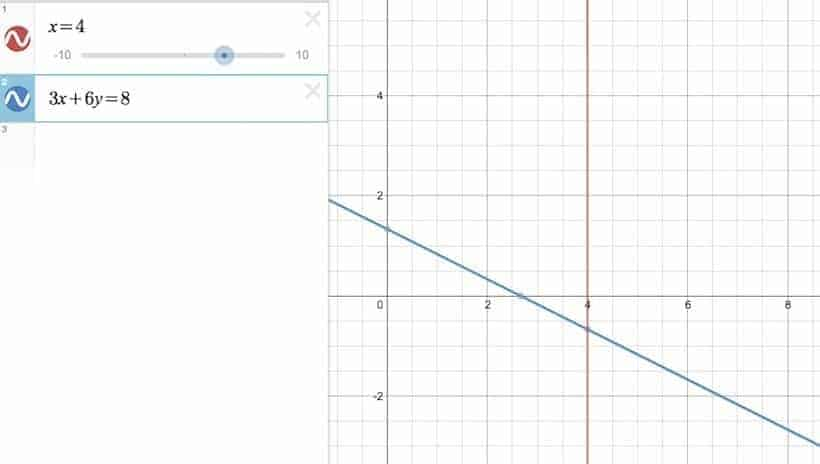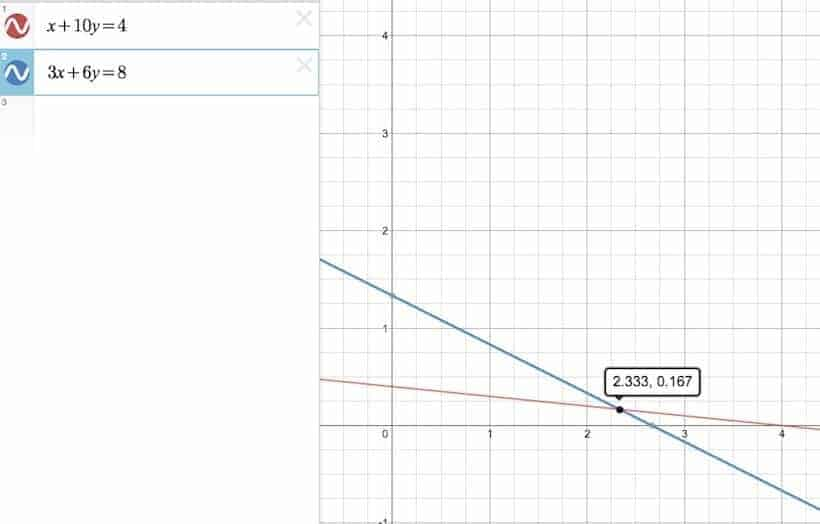# How to Solve a System of Linear Equations with an Additional Parameter?

College Math, Linear Algebra

(For more on matrices, vectors, vector products and other related topics, check out our Linear Algebra Ebook Series.)

Today, Connor asked the following question involving a system of linear equation with an additional parameter:

Given that $x_1 + hx_2 = 4$  and $3x_1 + 6x_2 =8$, find all pairs of the form $(x_1,x_2)$ satisfying both equations.

OK…What do we do with that additional $h$? Well, read on!

## Ignoring the h First

We are given this system of linear equations from the get-go:

\begin{cases}x_1+hx_2=4 & (a) \\ 3x_1+6x_2=8 & (b) \end{cases}

What to do? Well, we can try to solve this system of linear equations by regarding $h$ as an ordinary coefficient first, and see what it takes us to as we proceed judiciously. First, dividing the equation $(b)$ by $3$ on both sides yields the following equivalent system:

\begin{cases}x_1+hx_2=4 & (1) \\ x_1+2x_2=\frac{8}{3} & (2) \end{cases}

Turning the 2nd equation into $(2)-(1)$, we get:

\begin{align*} \begin{cases} x_1+hx_2=4 \\ (2-h)x_2=\frac{8}{3}-4 \end{cases} \qquad & \Leftrightarrow \qquad \begin{cases} x_1+hx_2=4 & (3) \\ (h-2)x_2=4-\frac{8}{3} = \frac{4}{3} & (4) \end{cases} \end{align*}

At this point, our latest system is still equivalent to the original one (i.e., $(a)$ and $(b) \iff (3)$ and $(4)$) . And with $(4)$, we are definitely one step closer towards solving for $x_2$. But before we do just that, notice that we can’t solve for $x_2$ by moving $h-2$ to the right, if $h-2=0$ (i.e., $h=2$). This would then open up two cans of worms…

## Case 1 — When h=2

Replace $h$ with 2 in the equations $(3)$ and $(4)$, the following system pops up:

\begin{cases} x_1+2x_2=4 \\ 0= \frac{4}{3} \end{cases}

Oops. Contradiction. That can’t happen at any rate, so there is no solution in this case.

In fact, we don’t even have to get to $(3)$ and $(4)$ to see it. This is because if we just plug in 2 into $h$ by the time we got to $(1)$ and $(2)$, we would get the following system:

\begin{cases} x_1+2x_2=4 \\ x_1+2x_2=\frac{8}{3} \end{cases}

which, as we can see, is already a system whose equations contradict each other. Here — a picture is worth a thousand words:

## Case 2 — When h ≠ 2

Once the above trivial hurdle is clear, we can then move on to something more serious. For the record, here is the latest system we have so far:

\begin{cases} x_1+hx_2=4 & (3)\\ (h-2)x_2=4 – \frac{8}{3} = \frac{4}{3} & (4) \end{cases}

Since $h \ne 2$ now, $h-2 \ne 0$. We can therefore proceed to divide the equation $(4)$ by $h-2$ on both sides, in which case we get:

\begin{cases} x_1+hx_2=4 & (5) \\ x_2= \frac{4}{3(h-2)} & (6) \end{cases}

Lastly, if we turn $(5)$ into $(5)-h(6)$, we get a very neat system in return:

\begin{cases} x_1=4 – \frac{4h}{3(h-2)}=\frac{8h-24}{3h-6} & (7) \\ x_2= \frac{4}{3(h-2)}= \frac{4}{3h-6}& (8) \end{cases}

So for each $h \ne 2$, we get a unique pair of solution of the form $\left( \dfrac{8h-24}{3h-6}, \dfrac{4}{3h-6} \right)$.

### Some Illustrations

For example, if $h=0$, then the original system becomes:

\begin{cases} x_1=4 \\ 3x_1+6x_2=8 \end{cases}

In which case, the unique solution would be:

$$\left( \frac{8h-24}{3h-6} , \frac{4}{3h-6} \right)_{h=0} = \left( 4,-\frac{2}{3} \right)$$

Here is a picture:The intersection point occurs at $(4, -\frac{2}{3})$.

And in the case where $h=10$, the original system becomes:

\begin{cases} x_1+10x_2=4 \\ 3x_1+6x_2=8 \end{cases}

In which case, the unique solution would be:

$$\left(\frac{8h-24}{3h-6}, \frac{4}{3h-6} \right)_{h=10} = \left(\frac{56}{24}, \frac{4}{24} \right) = \left( \frac{7}{3}, \frac{1}{6} \right)$$

Here is a picture again:Notice how the 1st equation (the red line) got rotated counter-clockwise about $(4,0)$ as h increases. The intersection point now occurs at $\displaystyle \left( \frac{7}{3}, \frac{1}{16} \right)$.

And this, is how we can solving infinitely many system of linear equations all at once! For a last sanity check, here’s a Desmos animation we’ve put together — just so that we can put everything we’ve learned thus far into a single slide.Math Vault